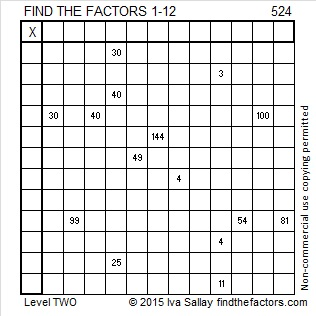# 524 and Level 2

24 is divisible by 4 and that means 524 is also divisible by 4.

Numbers that are divisible by 4 can have their square roots reduced. 524 ÷ 4 = 131, a prime number whose only square factor is 1, so √524 = (√4)(√131) = 2√131.Print the puzzles or type the solution on this excel file: 12 Factors 2015-06-15

—————————————————————————————————

• 524 is a composite number.
• Prime factorization: 524 = 2 x 2 x 131, which can be written 524 = (2^2) x 131
• The exponents in the prime factorization are 2 and 1. Adding one to each and multiplying we get (2 + 1)(1 + 1) = 3 x 2  = 6. Therefore 524 has exactly 6 factors.
• Factors of 524: 1, 2, 4, 131, 262, 524
• Factor pairs: 524 = 1 x 524, 2 x 262, or 4 x 131
• Taking the factor pair with the largest square number factor, we get √524 = (√4)(√131) = 2√131 ≈ 22.891046—————————————————————————————————